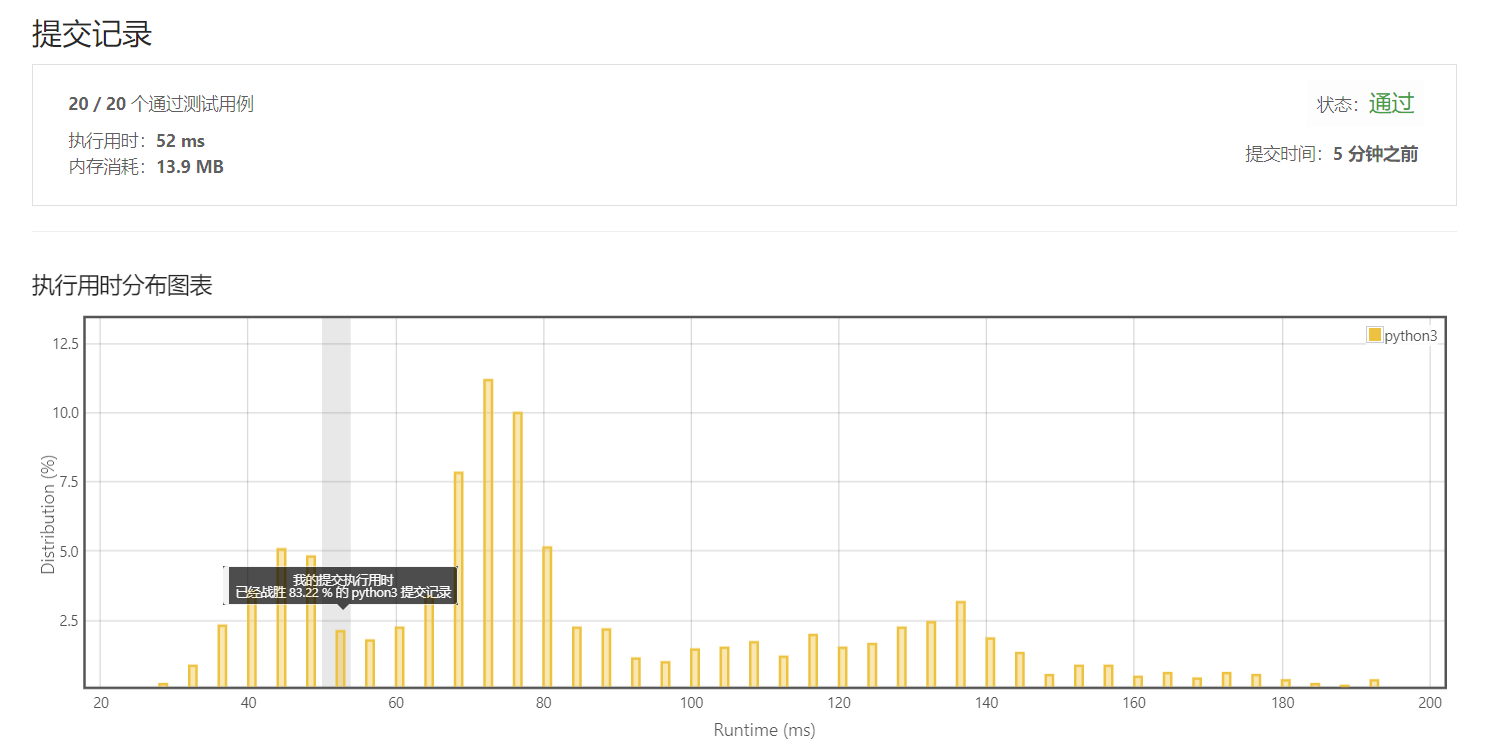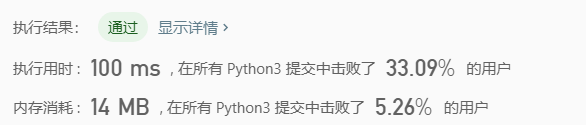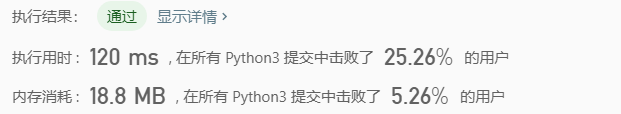• 分析:表达式求值一般都是模拟，但模拟的时候也有些小技巧，中缀表达式有一些优先级，受优先级的影响我们不能直接从前往后计算，这是我们就需要处理。我们可以借助两个栈，一个存操作符，另一个存操作数。具体操作见:...
题目链接：http://acm.nyist.net/JudgeOnline/problem.php?pid=35

题目大意：给你一个数学表达式，求出结果。

分析:表达式求值一般都是模拟，但模拟的时候也有些小技巧，中缀表达式有一些优先级，受优先级的影响我们不能直接从前往后计算，这是我们就需要处理。我们可以借助两个栈，一个存操作符，另一个存操作数。
具体算法分析见:中缀表达式变成等价的后缀表达式的算法，然后就是后缀表达式的求值，从前往后扫一遍即可；
具体执行操作见： 后缀表达式的求值的算法。在中缀变后缀的时候我们可以同时求后缀表达式（因为无优先级的影响）。

将中缀表达式转换为等价的后缀表达式的过程要使用一个栈放“（”，具体可以按照下面的方式进行。
（1）从左到右一次扫描中缀表达式的每一个字符，如果是数字字符和圆点“.”（可能是小数）则直接将它们写入后缀表达式中。
（2）如果遇到的是开括号“（”，则将它们压入一个操作符栈（不需要与栈顶操作符相比较），它表明一个新的计算层次的开始，在遇到和它匹配的闭括 号“）”时，将栈中的元素弹出来并放入后缀表达式中，直到栈顶元素为“(”时，将栈顶元素“（”弹出（不需要加入后缀表达式），表明这一层括号内的操作处 理完毕。
（3）如果遇到的是操作符，则将该操作符和操作符栈顶元素比较：
1、当所遇到的操作符的优先级小于或等于栈顶元素的优先级时，则取出栈顶元素放入后缀表达式，并弹出该栈顶元素，反复执行直到当前操作符的优先级大于栈顶元素的优先级；如果是在变后缀的时候同时求值，当所遇到的操作符的优先级小于或等于栈顶元素的优先级时，我们可以一直计算直到当前操作符的优先级大于栈顶元素的优先级或者操作符的栈为空。
2、当所遇到的操作符的优先级大于栈顶元素的优先级的时则将它压入栈中。
（4）重复上述步骤直到遇到中缀表达式的结束符标记“#”，弹出栈中的所有元素并放入后缀表达式中，转换结束

CODE：

#include<stdio.h>
#include<string.h>
#include<iostream>
#include<algorithm>
#include<stdlib.h>
#include<queue>
#include<stack>
#include<ctype.h>
#include<bits/stdc++.h>
using namespace std;
const int maxn=1005;
char s[maxn];
stack<double>n;
stack<char>op;
int getvalue(char c)//比较运算符的优先级
{
int ans;
if(c=='(')
ans=0;
if(c=='-'||c=='+')
ans=1;
if(c=='*'||c=='/')
ans=2;
return ans;
}
double cal(double a,double b,char c)
{
if(c=='+')
return a+b;
if(c=='-')
return a-b;
if(c=='*')
return a*b;
if(c=='/')
return a/b;
}
void pull()//分别取两个栈中的元素，计算表达式，后缀表达式无优先级，直接计算就行，最后数字栈中剩下的一个元素就是表达式的结果
{
double a,b;
if(n.size()>1&&!op.empty())
{
b=n.top();
n.pop();
a=n.top();
n.pop();
n.push(cal(a,b,op.top()));
op.pop();
}
}
int main()
{
int t;
scanf("%d",&t);
while(t--)
{
while(!n.empty()) n.pop();
while(!op.empty())  op.pop();
char ch;
double num;
scanf("%s",s);
int len=strlen(s);
for(int i=0; i<len;)
{
if(s[i]>='0'&&s[i]<='9')
{
//                sscanf(s+i,"%lf",&num);
//                n.push(num);
//                cout<<num<<"***"<<endl;
//                while((s[i]>='0'&&s[i]<='9')||s[i]=='.')
//                    i++;
double ans1=0,ans2=0,kk=0.1;
int flag=0;
while((s[i]>='0'&&s[i]<='9')||s[i]=='.')
{
//cout<<"***"<<endl;
if(s[i]=='.')
{
flag=1;
}
else
{
if(!flag)
ans1=ans1*10.0+(s[i]-'0')*1.0;
else
{
ans2=ans2+kk*(s[i]-'0');
kk*=0.1;
}
}
i++;
}
//cout<<ans1+ans2<<endl;
n.push(ans1+ans2);
}
ch=s[i++];
//cout<<ch<<"^^^^"<<endl;
if(ch=='=')
break;
if(ch=='(')
op.push(ch);
else if(ch==')')//如果遇到')'一直pull()操作，直到栈空或者遇到'('
{
while(!op.empty())
{
if(op.top()=='(')
{
op.pop();
break;
}
pull();
}
}
else
{
while(!op.empty()&&getvalue(ch)<=getvalue(op.top()))
pull();//比较栈顶和当前运算符的优先级，如果当前优先级小于栈顶的优先级，pull()操作
op.push(ch);
//cout<<ch<<"%%%"<<endl;
}
}
while(!op.empty())
pull();
printf("%.2lf\n",n.top());
}
}


展开全文• 表达式求值 实验11：整数算术表达式分析程序 题目类别: B作业 关键字: 类、对象、封装、类之间的关系、字符串 内容要求: 编写一个Java应用程序。 要求实现功能如下： (1) 从键盘输入一个表示整数...
表达式求值
实验11：整数算术表达式分析程序
题目类别: B作业
关键字: 类、对象、封装、类之间的关系、字符串
内容要求:

编写一个Java应用程序。

要求实现功能如下：

(1) 从键盘输入一个表示整数四则运算的字符串，例如：32+5*2-40/3
四则运算符号：
+代表整数加法、-代表整数减法、*代表整数乘法、/代表整数除法（结果为整数）
运算数全部为正整数
表达式中没有括号
假设输入的字符串一定是符合上面规则的整数四则运算的表达式。
运算数和运算符号之间可能有个数不定（0个或多个）的空格。
(2) 程序按照四则运算符的优先级和结合性对表达式进行分析并产生输出结果。

运行示例：

输入：32+5*2-40/3
输出：29
本次实验比较困扰我的有两个地方，1.如何将数字与运算符分隔开，2.中缀表达式的运算；


package ddd;
import java.util.Scanner;
import java.util.Stack;

public class calculation {

public static void main(String[] args) {
// TODO Auto-generated method stub
Scanner input = new Scanner(System.in);
System.out.println("请输入一个算术表达式: ");
String s = input.nextLine();//因为输入有空格，所以用nextline
String[] ss = s.split("\\b");//将数字和运算符分隔开

/*去掉每个字符串中的空格，但是replace并不会替代掉原来的内容
所以得赋值给自身
*/
for(int i=0;i<ss.length;i++) {
ss[i]=ss[i].replace(" ", "");
}

/*
分别用两个栈存放数字和运算符
用for-each检查每个字符串
当该字符串为数字时，直接进数字栈
当该字符串为运算符时：
判断运算符栈里有无运算符，若无则进栈。
若有运算符则判断两个运算符的优先级大小
如果当前运算符优先级>栈顶的运算符优先级，进栈
如果当前运算符优先级<栈顶的运算符优先级，取出数字栈里的两个字符串进行计算。
*/

Stack<Integer> number = new Stack<>();
Stack<String> fuhao = new Stack<>();

for(String sc : ss ) {
if(isOperator(sc)) {
int currOperLevel = judge(sc);//记录当前优先级;
while(true) {
int stackOperLevel = 0;
if(fuhao.isEmpty() == false) {
String dd = fuhao.peek();
stackOperLevel = judge(dd);
}
else {
fuhao.push(sc);
break;
}
if(currOperLevel > stackOperLevel) {
fuhao.push(sc);
break;
}
else {
String Optemp = "0";
int num1 = 0;
int num2 = 0;
if(fuhao.isEmpty() == false) {
Optemp = fuhao.pop();
}
if(number.isEmpty() == false) {
num1 = number.pop();
num2 = number.pop();

}
number.push(calculate(Optemp,num1,num2));
}
}
}
else {
int number1 = Integer.valueOf(sc);
number.push(number1);
}
}

//检查符号栈是否为空
while(true) {
if(fuhao.isEmpty() == false) {
String temp = "0";
temp = fuhao.pop();
int num1 = 0;
int num2 = 0;
if(number.isEmpty() == false) {
num1 = number.pop();
num2 = number.pop();

}
number.push(calculate(temp,num1,num2));
}
else
break;

}
System.out.println("结果为:"+number.pop());
input.close();

}

public static boolean youxj(String a,String b) {
int first = judge(a);
int second = judge(b);
if(first > second)
return true;
else
return false;
}

public static int judge(String o) {
int a = 0;
switch(o) {
case "+": {
a = 0;
break;
}
case "-": {
a = 0;
break;
}
case "*": {
a = 1;
break;
}
case "/": {
a = 1;
break;
}
}
return a;
}

public static int calculate(String c,int a,int b) {
int sum = 0;
switch(c) {
case "+":{
sum = a+b;
break;
}
case "-":{
sum = b-a;
break;
}
case "*":{
sum = a*b;
break;
}
case "/":{
sum = b/a;
break;
}
}
return sum;
}

public static boolean isOperator(String s) {
// 这里有一个需要注意的地方，就是字符串是传引用，不能直接做比较，
// 应该用eauals（）表示内容是否相同，  若为s=="+"，则表示对象相同。
if(s.equals("+")||s.equals("-")||s.equals("*")||s.equals("/"))
return true;
else
return false;
}
}





展开全文java
• 从键盘上输入一个后缀表达式，试编...逆波兰表达式(即后缀表达式)求值规则如下：设立运算数栈OPND,对表达式从左到右扫描(读入)，当表达式中扫描到数时，压入OPND栈。当扫描到运算符时，从OPND退出两个数，进行相应运...
从键盘上输入一个后缀表达式，试编写算法计算表达式的值。规定：逆波兰表达式的长度不超过一行，以$符作为输入结束，操作数之间用空格分隔,操作符只可能有+、-、*、/四种运算。例如：234 34+2 *$。
[题目分析]
逆波兰表达式(即后缀表达式)求值规则如下：设立运算数栈OPND,对表达式从左到右扫描(读入)，当表达式中扫描到数时，压入OPND栈。当扫描到运算符时，从OPND退出两个数，进行相应运算，结果再压入OPND栈。这个过程一直进行到读出表达式结束符$，这时OPND栈中只有一个数，就是结果。 #include<iostream> using namespace std; //节点结构体 typedef struct Node { int data; struct Node *next; }; //栈结构体 typedef struct Stack { struct Node *top; //栈顶指针 int size; //当前栈的大小 }; bool InitStack(Stack *&s) { s->top = NULL; if (s->top != NULL) return false; s->size = 0; return true; } bool push(Stack *&s, int data) { Node *newNode = new Node; newNode->data = data; newNode->next = s->top; s->top = newNode; s->size++; return true; } bool pop(Stack *&s,int &datat) { if (s->size == 0) return false; Node *p = s->top; s->top = p->next; delete p; s->size--; return true; } int GeTop(Stack *&s) { if (s->size == 0) return 0; return s->top->data; } bool StackEmpty(Stack *&s) { if (s->size == 0) return true; return false; } int In(char op) //判断是不是数字 { if (op == '0' || '1' || '2' || '3' || '4' || '5' || '6' || '7' || '8' || '9') return 1; return 0; } int change_to_int(char op) { return op - '0'; } int operate(int a1, int a2, char op) { switch (op) { case '+': return a1 + a2; break; case '-': return a1 - a2; break; case '*': return a1 * a2; break; case '/': return a1 / a2; break; } } void cal(Stack *&OPND) { char op; cout << "请输入操作数"; cin >> op; while (op != '$')
{
if (In(op)) //如果是操作数，直接放进栈中，并输入下一个
{
push(OPND, change_to_int(op));
cout << "请输入操作数";
cin >> op;
}
else //是操作符，两个操作数从栈中弹出
{
int a2 = 0, a1 = 0;
pop(OPND, a2);
pop(OPND, a1);
//将两个操作数取出来之后进行相应的运算。
int result=operate(a1, a2, op);
push(OPND, result);
cout << "请输入操作数";
cin >> op;
}
}
cout << "表达式计算的结果是:" << GeTop(OPND) << endl;
}

int main()
{
Stack *OPND = new Stack;
InitStack(OPND);
cal(OPND);

system("pause");
return 0;
}



展开全文• 根据逆波兰表示法，求表达式。 有效的运算符包括 +, -, *, / 。每个运算对象可以是整数，也可以是另一个逆波兰表达式。 说明： 整数除法只保留整数部分。 给定逆波兰表达式总是有效的。换句话说，表达式总会...
文章目录题目想法一：栈算法实现执行结果复杂度分析栈算法实现执行结果递归算法实现执行结果字典算法实现执行结果小结
题目
根据逆波兰表示法，求表达式的值。
有效的运算符包括 +, -, *, / 。每个运算对象可以是整数，也可以是另一个逆波兰表达式。
说明：

整数除法只保留整数部分。
给定逆波兰表达式总是有效的。换句话说，表达式总会得出有效数值且不存在除数为 0 的情况。

示例1 :
输入: ["2", "1", "+", "3", "*"]
输出: 9
解释: ((2 + 1) * 3) = 9

示例2 :
输入: ["2", "1", "+", "3", "*"]
输出: 9
解释: ((2 + 1) * 3) = 9

示例3 :
输入: ["10", "6", "9", "3", "+", "-11", "*", "/", "*", "17", "+", "5", "+"]
输出: 22
解释:
((10 * (6 / ((9 + 3) * -11))) + 17) + 5
= ((10 * (6 / (12 * -11))) + 17) + 5
= ((10 * (6 / -132)) + 17) + 5
= ((10 * 0) + 17) + 5
= (0 + 17) + 5
= 17 + 5
= 22

想法一：栈
算法实现
def evalRPN(self, tokens: List[str]) -> int:
stack = []
for i in tokens:
try:
stack.append(int(i))
except:
b = stack.pop()
a = stack.pop()
if i == "+":
stack.append(a + b)
elif i == "-":
stack.append(a - b)
elif i == "*":
stack.append(a * b)
elif i == "/":
stack.append(int(a / b))
return stack.pop()

执行结果
执行结果 : 通过
执行用时 : 52 ms, 在所有 Python3 提交中击败了83.22%的用户
内存消耗 : 13.9 MB, 在所有 Python3 提交中击败了5.26%的用户复杂度分析

时间复杂度：O(N)，这里 N 是字符串数量。
空间复杂度：O(N)

栈
算法实现
# 较为简洁，但比较慢，新函数eval()函数用来执行一个字符串表达式，并返回表达式的值。
def evalRPN(self, tokens: List[str]) -> int:
stack = []
for i in tokens:
if i in {'+', '-', '*', '/'}:
a, b = stack.pop(), stack.pop()
stack.append(str(int(eval(b + i + a))))
else:
stack.append(i)
return int(stack[-1])

执行结果递归
算法实现
def evalRPN(self, tokens: List[str]) -> int:
t, f = tokens.pop(), self.evalRPN
if t in '+-*/':
b, a = f(tokens), f(tokens)
t = eval('a' + t + 'b')
return int(t)

执行结果字典
算法实现
def evalRPN(self, tokens: List[str]) -> int:
tmp = []
op = {
"+": lambda x, y: x + y,
"-": lambda x, y: y - x,
"*": lambda x, y: x * y,
"/": lambda x, y: int(y / x)
}
for t in tokens:
if t in op:
r = tmp.append(op[t](tmp.pop(), tmp.pop()))
else:
tmp.append(int(t))
return tmp.pop()

执行结果小结
总的来说首先想到的是利用栈，不同做法的区别在于如何处理运算符的问题；然后说到栈肯定会想到递归，前者是后者的基本原理，所以可以互相转化，但是递归在这道题有些晦涩难懂，需要动手试试。
题虽简单，但是又学到了一些东西，比如eval()函数是用来执行一个字符串表达式，并返回表达式的值（再次感叹Python的强大）；而且学到了用字典也可以储存lambda函数，是个不错的方法。


展开全文字符串 python leetcode 算法
• 题目描述 根据逆波兰表示法，求表达式的值。 有效的运算符包括 +, -,...思路：波兰表达式又称后缀表达式，后缀表达式求值过程如下： 规则：从左到右遍历表达式的每个数字和符号，遇到数字就进栈，遇到字符就将栈顶的...leetcode c++ 数据结构
• 编制一个表达式求值的程序。 二、需求分析  本程序在Windows环境下用用Visual C++编写，要把一个表达式翻译成正确求值的一个机器指令序列，或者直接对表达式求值 首先要了解算术四则运算的规则。即：  1)先乘除，...实验 学习
• 一、文章来由按照惯例有这个section，但是木有什么可写的，一道笔试题而已~~~二、lisp表达式求值题目如下：分析： 初看题目，这就是一道很简单的ACM字符处理题，有点类似中缀表达式求值。但是字符题非常需要细心，...笔试 c++ 算法
• 1、题目：算术表达式求值 2、要求: （1）正确解释表达式； （2）符合四则运算规则： （3）先乘除、后加减； （4）从左到右运算； （5）先括号内，后括号外； （6）输出最后的计算结果。 二、问题描述 1、对一个合法...数据结构 c++
• 动态表达式求值 功能： (1) 输入并保存公式到文件。（公式中的操作数用字母代替，计算时给出具体值） (2) 将公式载入到表达式二叉树 (3) 输入操作数的具体值，计算结果 需求分析： 本演示程序中，表达式变量...
• 问题描述 : 以字符串形式输入仅有整数和加减（正负）号构成的表达式，输出该表达式。 输入说明 : 标准输入设备中有多组测试数据，每组输入数据由一行组成...题目分析表达式的输入为字符串的形式，通过字符数组接字符串 c++ 数据结构
• 数据结构实验二算术表达式求值实验报告 .doc 数据结构与数据库 实验报告 实验题目 算术表达式求值 学 院化学与材料科学学院 专业班级 09 级材料科学与工程系 PB0920603 姓 名维谷 学 号 PB09206285 邮 箱 ....
• 数据结构表达式求值实验报告，包括设计题目，需求分析，概要设计，详细设计， 测试结果，心得体会几个部分
• 题目: 给定一个基本的算术表达式，请编程计算表达式。 举例: 1*2 + 3*4 思路: Expression = "Term + Term" =======> evalTerm( "1*2" ) + evalTerm( "3*4" ) ; Term = "Factor * Facotr" =======> ...
• 题目分析： 利用栈的思想： 如果是数字，则压入栈；若为符号，即执行“pop取栈顶 -> pop取新栈顶 -> 计算”操作。 代码如下： class Solution: def evalRPN(self, tokens: List[str]) -> int: symbol=['+...leetcode python
• 根据逆波兰表示法，求表达式。 有效的运算符包括 +, -, *, / 。每个运算对象可以是整数，也可以是另一个逆波兰表达式。 说明： 整数除法只保留整数部分。 给定逆波兰表达式总是有效的。换句话说，表达式总会...
• 教师签名 嘉应学院 计算机学院 实验报告 课程名称 数据结构课程设计 开课学期 2017-2018学年第2学期 班 级 指导老师 实验题目 学生通讯录管理系统 学 号 姓 名 上机时间 (一) 需求分析 1输入的形式和输入的范围 ...
• 思路分析：逆波兰表达式求值 1、建一个栈。 2、利用增强for循环遍历，如果当前字符为数字，则压入栈中；如果当前字符为“+、-、* 、/”运算符，则弹出两个元素进行运算，并将运算结果压入栈中。 3、...leetcode
• 　表达式求值借助基本结构应该不用说了是栈，不管手写还是STL都没有太大关系。而中缀表达式最难控制的地方是优先级，算上+-*/^（）一共有四个优先级【+-，*/，， ^（）】（后面会提到一个三级的字符“负号”，这是...
• 读入一个只包含+，-，*，/，(，）的整数范围内的计算表达式，计算该表达式，输出的结构为double类型的一个（对于除法的运算是有可能带有小数的） 2. 思路分析： ① 首先这道题目使用常规的方法是很难正确...
• http://acm.hdu.edu.cn/showproblem.php?pid=1237 分析： 中缀表达式求值  题目中没有括号 代码里加了括号 首先知道运算符的优先关系 （图片来自网络）HDU 字符串处理
• 题目大意给你一个只包含0~ 9、+、*这 12 种字符的算式，出它的mod 10000分析题目有一个北大的神犇哥哥给我们讲过（我无耻的没有听懂），他说要用： 字符串 后缀表达式 栈 60多行的程序 Orz!%%%其实呢，简单的...
• 逆波兰表达式求值[Evaluate Reverse Polish Notation][中等]——分析及代码[Java]一、题目二、分析及代码1. 栈（1）思路（2）代码（3）结果三、其他 一、题目 根据 逆波兰表示法，求表达式的值。 有效的算符包括 +...LeetCode Java
• ## NOIP2013 表达式求值

千次阅读 2015-10-15 18:49:40
题目：...分析：栈的应用。两个栈，一个存数字一个存操作符，当遇到符号时计算优先级比它小的所有符号，则最后栈顶元素即为答案。 代码：#include #include #include #include using namespace s
• 利用栈来实现含有加，减，乘，除等基本运算，输出表达式(乘是字母x) 输入 3x(15/5)+8 输出 17 样例输入 Copy 24-[6+(27/3)x2] 样例输出 Copy 0 分析： 此题无疑多加了一个‘[]’。基本思路还是一样的，但是注意...C
• 我们先来分析一下 这里有三种不匹配的情况， 第一种情况，字符串里左方向的括号多余了 ，所以不匹配。 第二种情况，括号没有多余，但是 括号的类型没有匹配上。 第三种情况，字符串里右方向的括号多余了，所以不匹配...算法 leetcode 字符串
• 仔细分析题目，这就是一道模拟题…… 直接按照符号读入全部的数字，先算乘法，最后把全部数加起来就是结果了 记得要%10000取最后四位 【参考程序】 #include&lt;iostream&gt; #include&lt;cstring&...
• 可编辑范本 可编辑范本 }可编辑范本 } 可编辑范本 实习报告 题目 设计一个演示用运算优先法对算数表达式求值过程的程序 班级 姓名 学号 完成日期 一 需求分析 1建立运算数栈SqStack和运算符栈SqStack2辅助分析算符...
• 整式的化简常与求值相结合，解决此类问题的大致步骤为"一化、二代、三计算"，有时根据题目的特征和条件，灵活的选择解题方法，常见的类型有:一，直接代入求值1.已知a，b互为相反数，c，d互为倒数，|x|=1，求式子a+b......